# Star, an introduction to WYSIWYG editing

## Introduction

In this tutorial you will create a simple star component and write your own custom editor for it. You will learn to
• dynamically generate a mesh
• support WYSIWYG editing
• support editing in the scene view

This tutorial comes after the Custom List tutorial.

This tutorial is for Unity version 4.3 and above.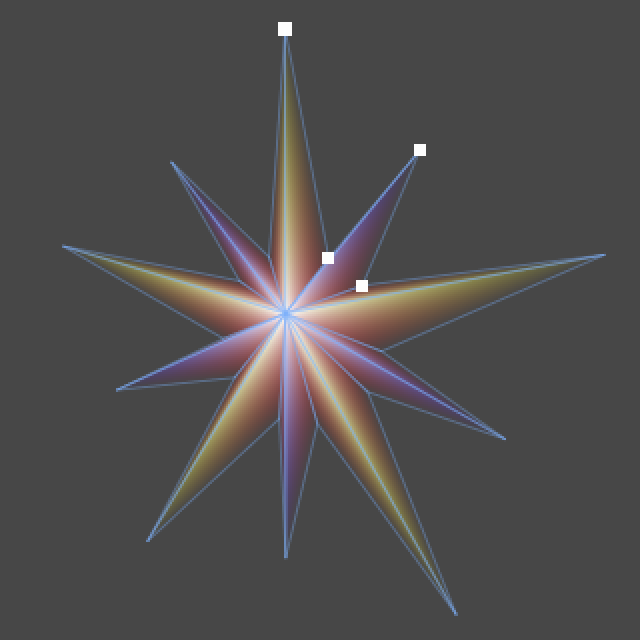WYSIWYG Star.

## Creating the star

We start with the finished Custom List tutorial project, or by creating a new empty project and importing custom-list.unitypackage.

We will be using the color point and editor list that we created previously, but we can get rid of all the testing code and objects.

The first thing we add is a C# script named Star. We'll use this script to create a circle made of triangles to produce a starlike effect, which requires a `Mesh`.

```using UnityEngine;

public class Star : MonoBehaviour {

private Mesh mesh;
}```
For this mesh to be of any use, it must be assigned to a `MeshFilter` component, which in turn is used by a `MeshRenderer` component. Only then will the mesh be drawn by Unity. So it is required that both these components are attached to the game object that our star component is also attached to.

Of course we can manually add these components, but we can also do this automatically by adding a `RequireComponent` attribute to our component.

```using UnityEngine;

[RequireComponent(typeof(MeshFilter), typeof(MeshRenderer))]
public class Star : MonoBehaviour {

private Mesh mesh;

}```
Now we create a new empty game object, name it My First Star, and add our component to. You will see that the object has also gained the other two components.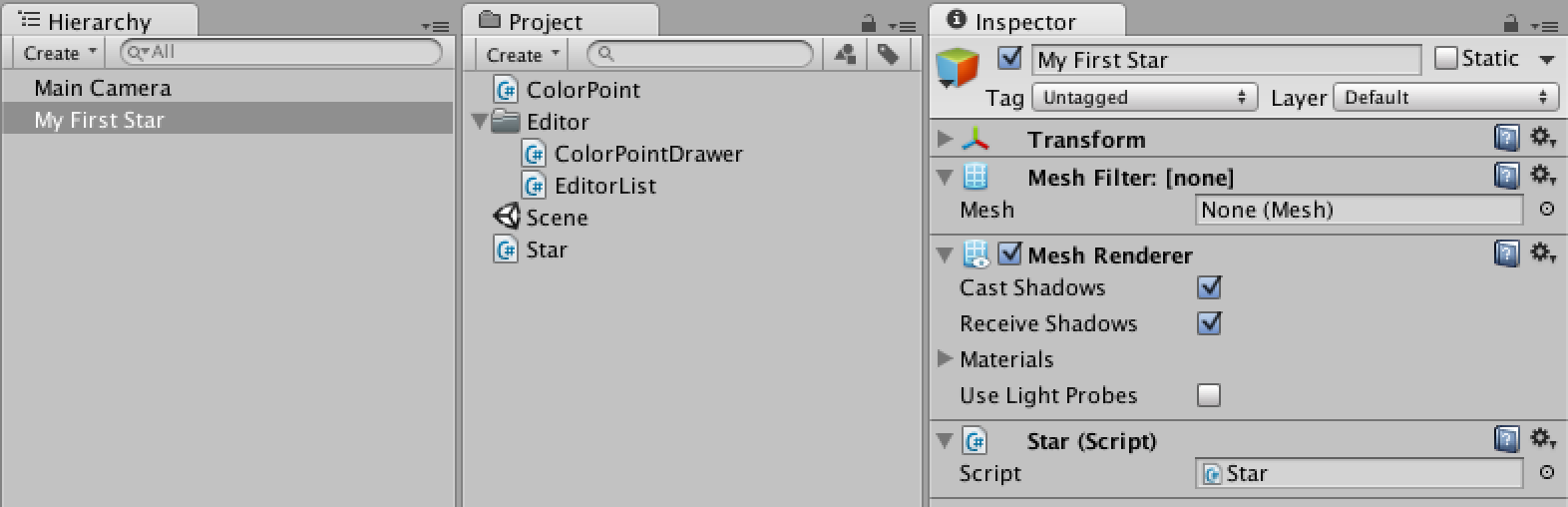Add one, get three.
The next step is to create a mesh. We'll do this in the `Start` Unity event method for now, so it happens as soon as we enter play mode. We also assign the mesh to the `MeshFilter` in one go and give it a descriptive name.
```	void Start () {
GetComponent<MeshFilter>().mesh = mesh = new Mesh();
mesh.name = "Star Mesh";
}```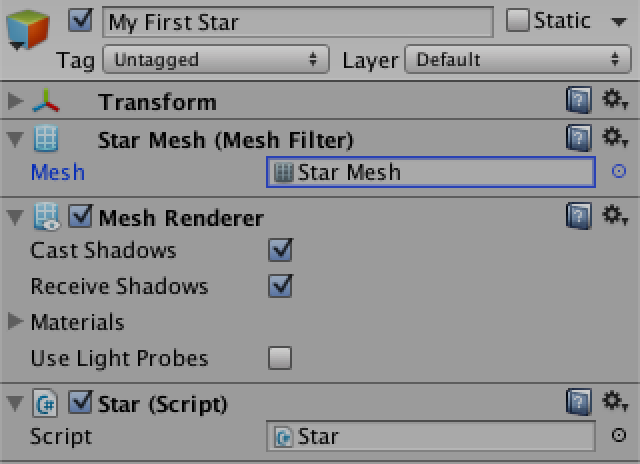A mesh appears in play mode.
Of course we won't see anything yet when entering play mode, because the mesh remains empty. So let's add an array of vertices, an option to control how many points our star has, and where these points should be placed relative to the center of the star.

The first vertex of our triangle fan sits at the center of the star, with all other vertices placed around it clockwise. We'll use a quaternion to rotate the points. The rotation angle is negative because we assume that we're looking down the Z axis, which makes positive rotation around Z go counterclockwise. We don't need to set the first vertex because vectors are set to zero by default.

```	public Vector3 point = Vector3.up;
public int numberOfPoints = 10;

private Mesh mesh;
private Vector3[] vertices;

void Start () {
GetComponent<MeshFilter>().mesh = mesh = new Mesh();
mesh.name = "Star Mesh";

vertices = new Vector3[numberOfPoints + 1];
float angle = -360f / numberOfPoints;
for(int v = 1; v < vertices.Length; v++){
vertices[v] = Quaternion.Euler(0f, 0f, angle * (v - 1)) * point;
}

mesh.vertices = vertices;
}```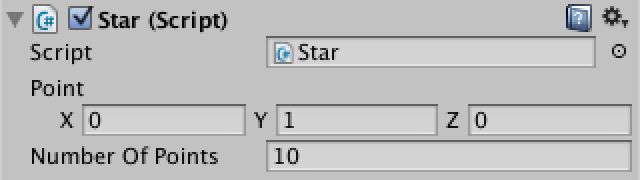Some points.
Triangles are stored as an array of vertex indices, three per triangle. Because we're using a triangle fan approach, every triangle starts at the first vertex and connects with the previous and next triangle. The last triangle wraps back to the first one. Fox example, if we had four triangles, the vertex indices would be `{0, 1, 2, 0, 2, 3, 0, 3, 4, 0, 4, 1}`.
```	private int[] triangles;

void Start () {
GetComponent<MeshFilter>().mesh = mesh = new Mesh();
mesh.name = "Star Mesh";

vertices = new Vector3[numberOfPoints + 1];
triangles = new int[numberOfPoints * 3];
float angle = -360f / numberOfPoints;
for(int v = 1, t = 1; v < vertices.Length; v++, t += 3){
vertices[v] = Quaternion.Euler(0f, 0f, angle * (v - 1)) * point;
triangles[t] = v;
triangles[t + 1] = v + 1;
}
triangles[triangles.Length - 1] = 1;

mesh.vertices = vertices;
mesh.triangles = triangles;
}```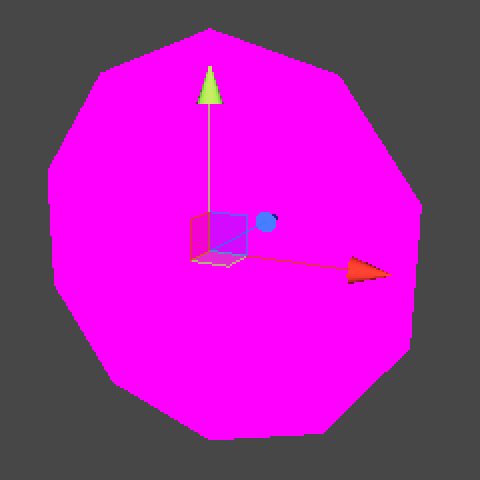One ugly star.
Right now our star looks like a very ugly polygon. Unity also complains about missing texture coordinates because the default shader expects them. As we won't be using a texture at all, let's get rid of that warning by creating our own shader that only uses vertex colors.

Create a new shader asset and name it Star, then put the following code in it.

```Shader "Star" {
SubShader {
Tags { "Queue"="Transparent" "IgnoreProjector"="True" "RenderType"="Transparent" }
Blend SrcAlpha OneMinusSrcAlpha
Cull Off
Lighting Off
ZWrite Off
Pass {
CGPROGRAM
#pragma vertex vert
#pragma fragment frag

struct data {
float4 vertex : POSITION;
fixed4 color: COLOR;
};

data vert (data v) {
v.vertex = mul(UNITY_MATRIX_MVP, v.vertex);
return v;
}

fixed4 frag(data f) : COLOR {
return f.color;
}
ENDCG
}
}
}```
Now we create a new material named – you guessed it – Star, set its shader to the one we just created, and drag it onto My First Star.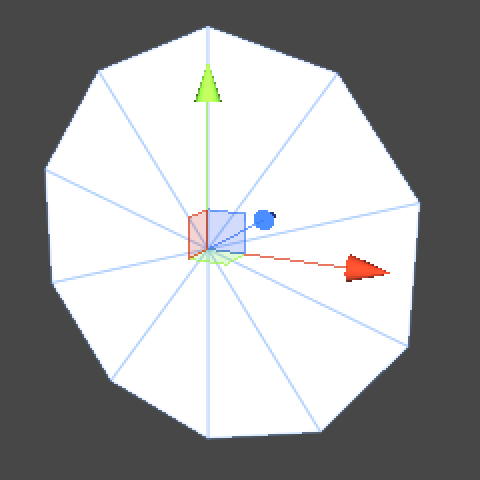A white star.
Vertex colors are white by default, so now our polygon turns up white. However, we want a multicolored star with points at varying distances from the center. So instead of configuring a single point, let's configure an array of points instead.

Let's also add a frequency option so we can automatically repeat point sequences instead of having to configure every single point of the star. This option replaces `numberOfPoints`.

We also include a check to make sure that the frequency is at least 1, because anything less wouldn't make any sense. And finally it is only possible to construct a mesh when we have at leasts three points.

```	public Vector3[] points;
public int frequency = 1;

void Start () {
GetComponent<MeshFilter>().mesh = mesh = new Mesh();
mesh.name = "Star Mesh";

if (frequency < 1) {
frequency = 1;
}
if (points == null) {
points = new Vector3;
}
int numberOfPoints = frequency * points.Length;
vertices = new Vector3[numberOfPoints + 1];
triangles = new int[numberOfPoints * 3];

if (numberOfPoints >= 3) {
float angle = -360f / numberOfPoints;
for(int repetitions = 0, v = 1, t = 1; repetitions < frequency; repetitions++){
for(int p = 0; p < points.Length; p += 1, v += 1, t += 3){
vertices[v] = Quaternion.Euler(0f, 0f, angle * (v - 1)) * points[p];
triangles[t] = v;
triangles[t + 1] = v + 1;
}
}
triangles[triangles.Length - 1] = 1;
}
mesh.vertices = vertices;
mesh.triangles = triangles;
}```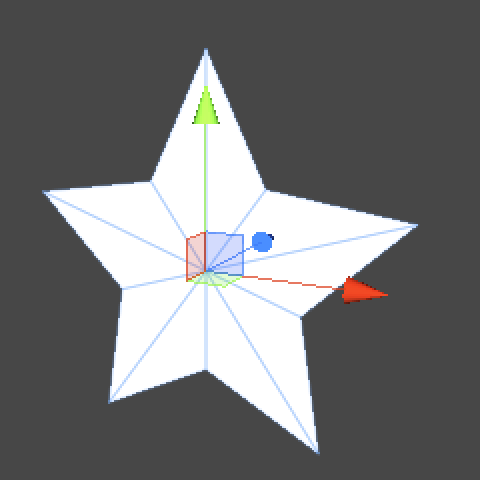Now with pointy points.
It's time to add some color! We can do this quite easily by using our `ColorPoint` data structure instead of just vectors for our points array. Let's also use a color point to define the center of the star, so we could move it around as well.
```	public ColorPoint center;
public ColorPoint[] points;

private Color[] colors;

void Start () {
GetComponent<MeshFilter>().mesh = mesh = new Mesh();
mesh.name = "Star Mesh";

if (frequency < 1) {
frequency = 1;
}
if (points == null) {
points = new ColorPoint;
}
int numberOfPoints = frequency * points.Length;
vertices = new Vector3[numberOfPoints + 1];
colors = new Color[numberOfPoints + 1];
triangles = new int[numberOfPoints * 3];

if (numberOfPoints >= 3) {
vertices = center.position;
colors = center.color;
float angle = -360f / numberOfPoints;
for(int repetitions = 0, v = 1, t = 1; repetitions < frequency; repetitions++){
for(int p = 0; p < points.Length; p += 1, v += 1, t += 3){
vertices[v] = Quaternion.Euler(0f, 0f, angle * (v - 1)) * points[p].position;
colors[v] = points[p].color;
triangles[t] = v;
triangles[t + 1] = v + 1;
}
}
triangles[triangles.Length - 1] = 1;
}
mesh.vertices = vertices;
mesh.colors = colors;
mesh.triangles = triangles;
}```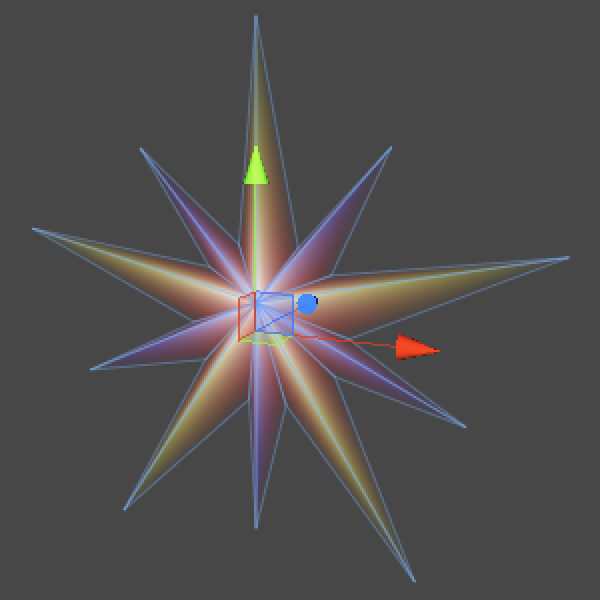Now with colored points.
If you see nothing when entering play mode after this step, try adjusting the alpha component of the colors. I've set the center color to fully opaque and left all other points fully transparent, resulting in a fade from the center to the edge.

## Improving the Inspector

Now that we can make nice stars, let's focus on our component's inspector. Thanks to the property drawer of `ColorPoint` it's looking decent, but we can improve it by creating a custom editor.

Create a new C# script named StarInspector in the Editor folder. Make it a custom editor with a straightforward GUI method, just like we did in the Custom List tutorial.

```using UnityEditor;
using UnityEngine;

[CustomEditor(typeof(Star)), CanEditMultipleObjects]
public class StarInspector : Editor {

public override void OnInspectorGUI () {
serializedObject.Update();
EditorGUILayout.PropertyField(serializedObject.FindProperty("center"));
EditorGUILayout.PropertyField(serializedObject.FindProperty("points"), true);
EditorGUILayout.PropertyField(serializedObject.FindProperty("frequency"));
serializedObject.ApplyModifiedProperties();
}
}```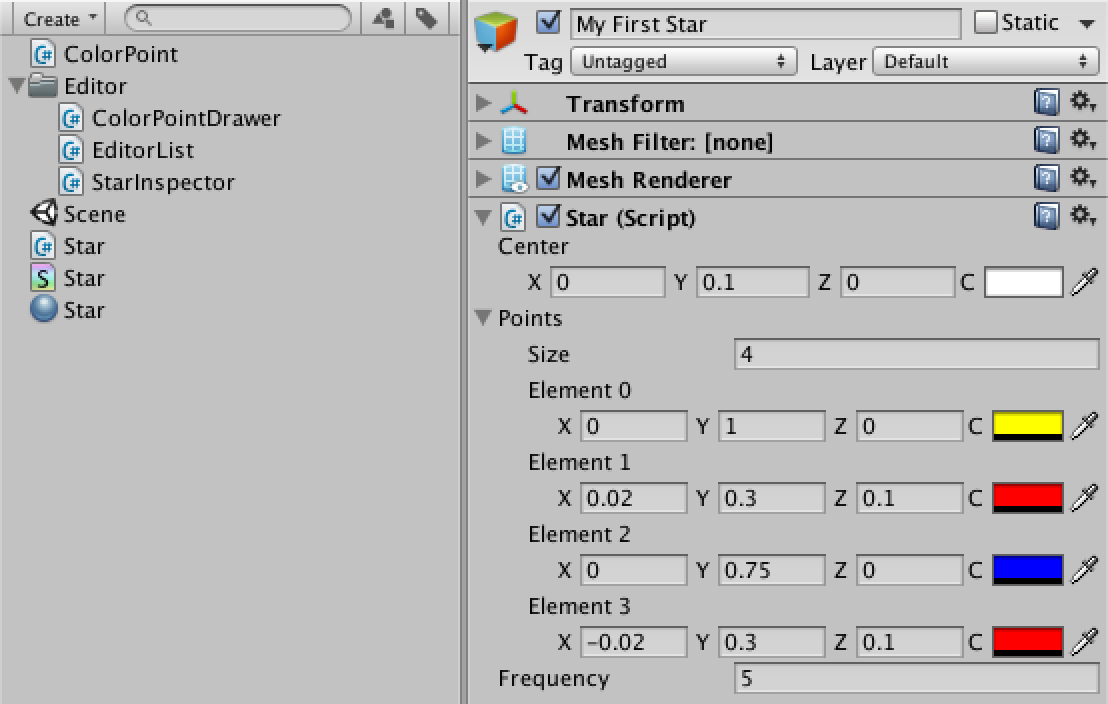Custom editor.
Now let's improve this layout by using our `EditorList` to display the points more compact and with button. Let's also use an integer slider with a range of 1–20 for the frequency.
```	public override void OnInspectorGUI () {
serializedObject.Update();
EditorGUILayout.PropertyField(serializedObject.FindProperty("center"));
EditorList.Show(
serializedObject.FindProperty("points"),
EditorListOption.Buttons | EditorListOption.ListLabel);
EditorGUILayout.IntSlider(serializedObject.FindProperty("frequency"), 1, 20);
serializedObject.ApplyModifiedProperties();
}```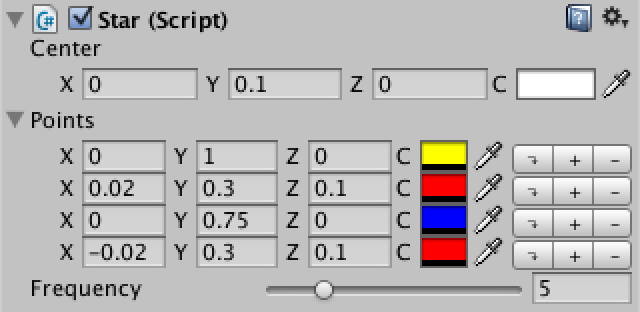Improved inspector.
As an additional feature, we could display how many points our star has, or give a warning when there are not enough points.
```	public override void OnInspectorGUI () {
SerializedProperty
points = serializedObject.FindProperty("points"),
frequency = serializedObject.FindProperty("frequency");
serializedObject.Update();
EditorGUILayout.PropertyField(serializedObject.FindProperty("center"));
EditorList.Show(points, EditorListOption.Buttons | EditorListOption.ListLabel);
EditorGUILayout.IntSlider(frequency, 1, 20);
int totalPoints = frequency.intValue * points.arraySize;
if (totalPoints < 3) {
EditorGUILayout.HelpBox("At least three points are needed.", MessageType.Warning);
}
else {
EditorGUILayout.HelpBox(totalPoints + " points in total.", MessageType.Info);
}
star.ApplyModifiedProperties();
}```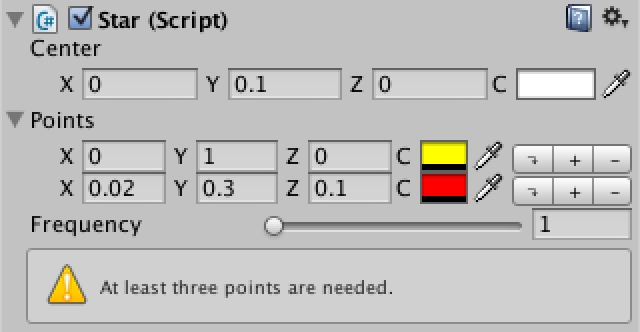Extra info.

## WYSIWYG

While our inspector is pretty good at this point, it's a bummer we can't see the star while we're editing it. It's about time we change that!

The first thing we need to do is tell Unity that our component should be active in edit mode. We indicate this by adding the `ExecuteInEditMode` class attribute. From now on, our `Start` method will be called whenever a star manifests in the editor.

Because we create a mesh in `Start`, it will be created in edit mode. As we assign it to a `MeshFilter`, it will persist and be saved in the scene. We don't want this to happen, because we generate the mesh dynamically. We can prevent Unity from saving the mesh by settings the appropriate `HideFlags`.

```using UnityEngine;

[ExecuteInEditMode, RequireComponent(typeof(MeshFilter), typeof(MeshRenderer))]
public class Star : MonoBehaviour {

public ColorPoint center;
public ColorPoint[] points;
public int frequency = 1;

private Mesh mesh;
private Vector3[] vertices;
private Color[] colors;
private int[] triangles;

void Start () {
GetComponent<MeshFilter>().mesh = mesh = new Mesh();
mesh.name = "Star Mesh";
mesh.hideFlags = HideFlags.HideAndDontSave;

if (frequency < 1) {
frequency = 1;
}
if (points == null) {
points = new ColorPoint;
}
int numberOfPoints = frequency * points.Length;
vertices = new Vector3[numberOfPoints + 1];
colors = new Color[numberOfPoints + 1];
triangles = new int[numberOfPoints * 3];

if (numberOfPoints >= 3) {
vertices = center.position;
colors = center.color;
float angle = -360f / numberOfPoints;
for(int repetitions = 0, v = 1, t = 1; repetitions < frequency; repetitions++){
for(int p = 0; p < points.Length; p += 1, v += 1, t += 3){
vertices[v] = Quaternion.Euler(0f, 0f, angle * (v - 1)) * points[p].position;
colors[v] = points[p].color;
triangles[t] = v;
triangles[t + 1] = v + 1;
}
}
triangles[triangles.Length - 1] = 1;
}
mesh.vertices = vertices;
mesh.colors = colors;
mesh.triangles = triangles;
}
}```
Now when the editor redraws – you can click or save the scene to trigger this – our star will show up in editor mode! However, it won't change when we modify the star component. That's because `Start` only gets called the first time the component is activated. Let's move the code to its own public method so we can explicitly call it whenever we want to. We'll also add a few checks that prevent recreation of the mesh and the arrays if that's not needed.

Finally, because we're reusing the mesh, we should clear it when the amount of vertices changes before assigning new data to it. Otherwise it will complain about a mismatch.

```	void Start () {
UpdateMesh();
}

public void UpdateMesh () {
if (mesh == null) {
GetComponent<MeshFilter>().mesh = mesh = new Mesh();
mesh.name = "Star Mesh";
mesh.hideFlags = HideFlags.HideAndDontSave;
}

if (frequency < 1) {
frequency = 1;
}
if (points == null) {
points = new ColorPoint;
}
int numberOfPoints = frequency * points.Length;
if (vertices == null || vertices.Length != numberOfPoints + 1) {
vertices = new Vector3[numberOfPoints + 1];
colors = new Color[numberOfPoints + 1];
triangles = new int[numberOfPoints * 3];
mesh.Clear();
}

if (numberOfPoints >= 3) {
vertices = center.position;
colors = center.color;
float angle = -360f / numberOfPoints;
for(int repetitions = 0, v = 1, t = 1; repetitions < frequency; repetitions++){
for(int p = 0; p < points.Length; p += 1, v += 1, t += 3){
vertices[v] = Quaternion.Euler(0f, 0f, angle * (v - 1)) * points[p].position;
colors[v] = points[p].color;
triangles[t] = v;
triangles[t + 1] = v + 1;
}
}
triangles[triangles.Length - 1] = 1;
}
mesh.vertices = vertices;
mesh.colors = colors;
mesh.triangles = triangles;
}```
In our editor, the `ApplyModifiedProperties` method returns whether any modifications were actually made. If so, we should call the `UpdateStar` method of the changed stars. The editor contains a `targets` array with all the currently selected `Star` components, so we can iterate through that.
```	public override void OnInspectorGUI () {
SerializedProperty
points = serializedObject.FindProperty("points"),
frequency = serializedObject.FindProperty("frequency");
serializedObject.Update();
EditorGUILayout.PropertyField(serializedObject.FindProperty("center"));
EditorList.Show(points, EditorListOption.Buttons | EditorListOption.ListLabel);
EditorGUILayout.IntSlider(frequency, 1, 20);
int totalPoints = frequency.intValue * points.arraySize;
if (totalPoints < 3) {
EditorGUILayout.HelpBox("At least three points are needed.", MessageType.Warning);
}
else {
EditorGUILayout.HelpBox(totalPoints + " points in total.", MessageType.Info);
}
if (serializedObject.ApplyModifiedProperties()) {
foreach (Star s in targets) {
s.UpdateMesh();
}
}
}```
Now the mesh gets updated immediately after we make a modification. This makes editing a whole lot easier! Alas, it does not respond to undo!

Unfortunately, there's no easy universal guaranteed way to detect undo events in Unity, but we can get pretty close. In our case, we can suffice by checking whether a `ValidateCommand` event happened that refers to an undo action. As this event must relate to the currently selected object, we just assume it was our component that got modified.

```	public override void OnInspectorGUI () {
SerializedProperty
points = serializedObject.FindProperty("points"),
frequency = serializedObject.FindProperty("frequency");
serializedObject.Update();
EditorGUILayout.PropertyField(serializedObject.FindProperty("center"));
EditorList.Show(points, EditorListOption.Buttons | EditorListOption.ListLabel);
EditorGUILayout.IntSlider(frequency, 1, 20);
int totalPoints = frequency.intValue * points.arraySize;
if (totalPoints < 3) {
EditorGUILayout.HelpBox("At least three points are needed.", MessageType.Warning);
}
else {
EditorGUILayout.HelpBox(totalPoints + " points in total.", MessageType.Info);
}
if (serializedObject.ApplyModifiedProperties() ||
(Event.current.type == EventType.ValidateCommand &&
Event.current.commandName == "UndoRedoPerformed")) {
foreach (Star s in targets) {
s.UpdateMesh();
}
}
}```
Finally, sweet editing! Anything else? Well, ever reset a component? At the top right of each component's inspector sits a gear icon with an option to reset that component. Sure enough, our mesh does not get updated when you reset the star component.

We can detect a component reset by adding a `Reset` method to `Star` component. This is a Unity event method that is only used inside the editor. Whenever this event happens, all we need to do is update the mesh.

```	void Reset () {
UpdateMesh();
}```
Ok, now resetting works too. Are we done? Well, what about prefabs?

Granted, it doesn't make much sense to use prefabs for our star, because each star generates its own little mesh. If you wanted to use lots of similar stars, it would be a better idea to create a star model in a 3D editor and import the mesh. That way all the stars can share the same mesh. But suppose we do want to support using a prefab, just to instantiate similar stars that we might later tweak individually.

Because prefabs don't exist as instances in the scene, we do not want to create a mesh for them. Their Unity event methods will never be called, but we would still update it ourselves in our editor. We can use the `PrefabUtility.GetPrefabType` method to detect whether our inspector target is a prefab. If so, we won't update it.

```	public override void OnInspectorGUI () {
SerializedProperty
points = serializedObject.FindProperty("points"),
frequency = serializedObject.FindProperty("frequency");
serializedObject.Update();
EditorGUILayout.PropertyField(serializedObject.FindProperty("center"));
EditorList.Show(points, EditorListOption.Buttons | EditorListOption.ListLabel);
EditorGUILayout.IntSlider(frequency, 1, 20);
int totalPoints = frequency.intValue * points.arraySize;
if (totalPoints < 3) {
EditorGUILayout.HelpBox("At least three points are needed.", MessageType.Warning);
}
else {
EditorGUILayout.HelpBox(totalPoints + " points in total.", MessageType.Info);
}
if (serializedObject.ApplyModifiedProperties() ||
(Event.current.type == EventType.ValidateCommand &&
Event.current.commandName == "UndoRedoPerformed")) {
foreach (Star s in targets) {
if (PrefabUtility.GetPrefabType(s) != PrefabType.Prefab) {
s.UpdateMesh();
}
}
}
}```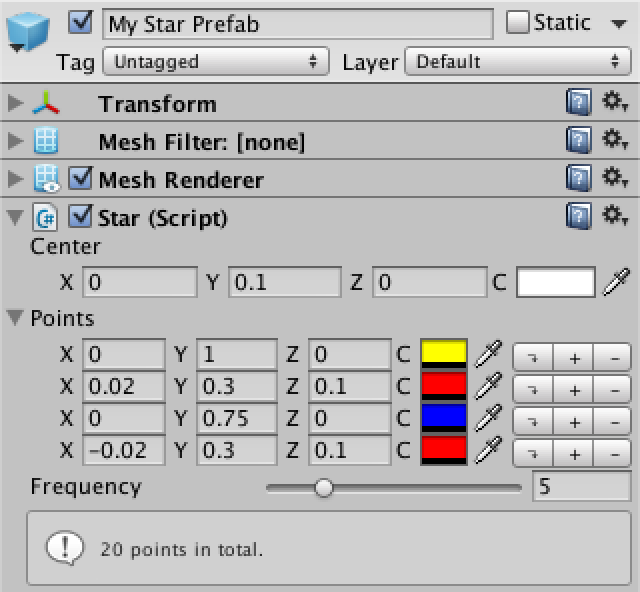Star prefab.
Now we can safely drag a star from the hierarchy into the project view to turn it into a prefab.

Unfortunately, modifications to the prefab do not cause an update of the mesh of the prefab instance. It turns out that every prebab modification triggers the `OnDisable` and `OnEnable` Unity event methods of all their instances. We can use this to update our mesh. And because `OnEnable` is also always called when an object becomes active, we can simply replace our `Start` method with `OnEnable`. Now changes to the prefab will get picked up by their instances.

```	void OnEnable () {
UpdateMesh();
}```
Another little detail is multi-object editing. While that works fine, it doesn't make much sense to show the point total, because this needn't be the same for the entire selection. So let's only show it when we have a single-object selection.
```	public override void OnInspectorGUI () {
SerializedProperty
points = serializedObject.FindProperty("points"),
frequency = serializedObject.FindProperty("frequency");
serializedObject.Update();
EditorGUILayout.PropertyField(serializedObject.FindProperty("center"));
EditorList.Show(points, EditorListOption.Buttons | EditorListOption.ListLabel);
EditorGUILayout.IntSlider(frequency, 1, 20);
if (!serializedObject.isEditingMultipleObjects) {
int totalPoints = frequency.intValue * points.arraySize;
if (totalPoints < 3) {
EditorGUILayout.HelpBox("At least three points are needed.", MessageType.Warning);
}
else {
EditorGUILayout.HelpBox(totalPoints + " points in total.", MessageType.Info);
}
}
if (serializedObject.ApplyModifiedProperties() ||
(Event.current.type == EventType.ValidateCommand &&
Event.current.commandName == "UndoRedoPerformed")) {
foreach (Star s in targets) {
if (PrefabUtility.GetPrefabType(s) != PrefabType.Prefab) {
s.UpdateMesh();
}
}
}
}```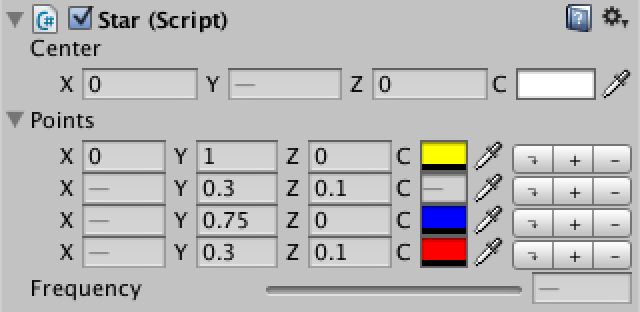Multi-object editing.

## Editing in the Scene View

Now we do have a nice inspector indeed, but wouldn't it be cool if we could edit the points directly in the scene view? By adding the `OnSceneGUI` Unity event method to our inspector, we can. This method will be called once per selected object, during which that object will be assigned to the `target` variable. We shouldn't use our `SerializedObject` here. In fact, it's best to think of this method as being completely separate from the rest of our editor.
```	void OnSceneGUI () {
}```
Let's put a small square handle at the top of the star's points. We only do this the first time a point appears, not for all their repetitions due to frequency. Placing these points works just like generating the star's mesh, except that we're working in world space here, not local space, so we need to apply the star's transformation.

We'll use the `Handles.FreeMoveHandle` method to draw our handles, which has a couple of parameters. First, it needs the position – in world space – for the handle. Then it needs the rotation of the handle, which we'll just leave unrotated. Next it wants the size of the handle, we'll use a small value here that looks good. Then comes a vector used for the snapping size (hold Control or Command to snap), which we configure as (0.1, 0.1 0.1). The last parameter is used to define the shape of the handle.

```	private static Vector3 pointSnap = Vector3.one * 0.1f;

void OnSceneGUI () {
Star star = target as Star;
Transform starTransform = star.transform;

float angle = -360f / (star.frequency * star.points.Length);
for (int i = 0; i < star.points.Length; i++) {
Quaternion rotation = Quaternion.Euler(0f, 0f, angle * i);
Vector3 oldPoint = starTransform.TransformPoint(rotation * star.points[i].position);
Handles.FreeMoveHandle(oldPoint, Quaternion.identity, 0.02f, pointSnap, Handles.DotCap);
}
}```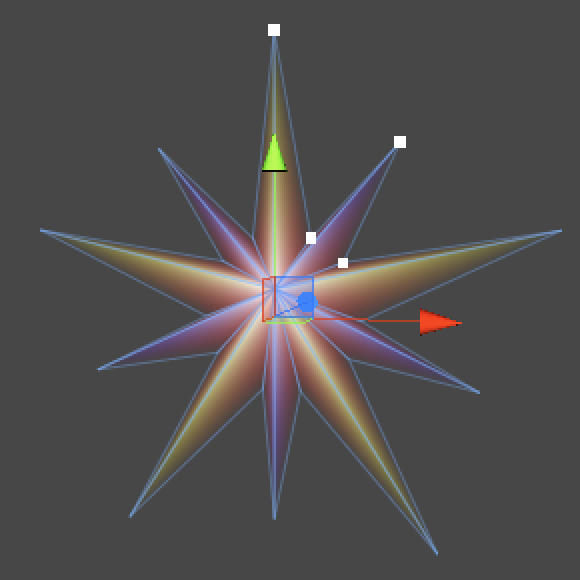Extra points for the scene view.
We now have nice handles that don't do much yet. You can click one and it becomes yellow, that's it. What we need to do is compare the position we put into the handle with the position that the handle returns. If they differ, the user dragged the handle and we should modify the star. We shouldn't forget to convert the new position back to the star's local space before assigning it to the point's offset and updating the star.
```	void OnSceneGUI () {
Star star = target as Star;
Transform starTransform = star.transform;

float angle = -360f / (star.frequency * star.points.Length);
for (int i = 0; i < star.points.Length; i++) {
Quaternion rotation = Quaternion.Euler(0f, 0f, angle * i);
Vector3
oldPoint = starTransform.TransformPoint(rotation * star.points[i].position),
newPoint = Handles.FreeMoveHandle(
oldPoint, Quaternion.identity, 0.02f, pointSnap, Handles.DotCap);
if (oldPoint != newPoint) {
star.points[i].position = Quaternion.Inverse(rotation) *
starTransform.InverseTransformPoint(newPoint);
star.UpdateMesh();
}
}
}```
Yes, it works! Wait, it doesn't support undo! We can't rely on `SerializedObject` here, but fortunately the handles can take care of the undo stuff for us. All we need to do is tell them which object is being edited and how the undo step should be named. We can do that with the `Undo.RecordObject` method.
```	void OnSceneGUI () {
Star star = target as Star;
Transform starTransform = star.transform;

float angle = -360f / (star.frequency * star.points.Length);
for (int i = 0; i < star.points.Length; i++) {
Quaternion rotation = Quaternion.Euler(0f, 0f, angle * i);
Vector3
oldPoint = starTransform.TransformPoint(rotation * star.points[i].position),
newPoint = Handles.FreeMoveHandle(
oldPoint, Quaternion.identity, 0.02f, pointSnap, Handles.DotCap);
if (oldPoint != newPoint) {
Undo.RecordObject(star, "Move");
star.points[i].position = Quaternion.Inverse(rotation) *
starTransform.InverseTransformPoint(newPoint);
star.UpdateMesh();
}
}
}```
And with that, we're done! Have fun designing stars!

## Downloads

star.unitypackage
The finished project.

# Questions & Answers

What's a `Mesh`?
Conceptually, a `Mesh` is a construct used by the graphics hardware to draw complex stuff. It contains at least a collection of points in 3D space plus a set of triangles – the most basic 2D shapes – defined by these points. The triangles constitute the surface of whatever the mesh respresents. Often, you won't realize that you're looking at a bunch of triangles instead of a real object.
What's with the weird `for` loop?
You can put multiple statements in the iterator declaration and increment parts of a `for` construct. The only weird thing is that you have to separate the statements with a comma in both places.

`for(int iA = 0, iB = 0; iA < 10; iA++, iB++) { DoStuff(iA, iB); }`

does the same as

`int iA = 0, iB = 0; while(iA < 10) { DoStuff(iA++, iB++); }`

What does the CGPROGRAM do?
Basically, data flows from the Unity engine into the graphics card, where it's processed per vertex. Then interpolated data flows from the vertices down to the individual pixels. In this case, we pass position and color data all the way down. The only additional thing we do is convert vertex positions from world space to screen space.

The statements above the CGPROGRAM switch off default lighting and depth buffer writing. Culling is switched off so we can see the triangles from both sides, not just the front. "Blend SrcAlpha OneMinusSrcAlpha" is default alpha blending, allowing for transparency.

Why no fixed function shader?
Fixed function shaders belong to the past. Also, the CGPROGRAM makes it more obvious how data from Unity is transformed into screen pixels.
Why check for `null`?
When freshly created, our star component won't have an array yet. It's also technically possible for scripts to explicitly set our array to `null`. We need to watch out for that, to prevent errors.
What does `foreach` do?
`foreach` is a convenient alternative for a `for` loop. Because it has some overhead compared to a regular `for` loop, I never use it in game code. But I don't have such reservations for using it in editor code if I don't need the iterator integer.

`foreach(Star s in targets) { s.UpdateStar(); }`

does the same as

`for(int i = 0; i < targets.Length; i++) { (targets[i] as Star).UpdateStar(); }`

An additional benefit of `foreach` is that it performs an implicit cast, so we don't need to write it ourselves.

What's a `ValidateCommand`?
`ValidateCommand` is a type of GUI event, which indicates that some special action happened, like undo or redo. So why isn't it called something like `ExecuteCommand`? Actually, that command type exists as well. While they have a slightly different meaning, in practice you use them for the exact same purpose. Unfortunately, depening on exactly where you're checking and how you're constructing your GUI, either one or the other event happens, but not both. Why this is so, I do not know.

So to be perfectly safe, you have to check for both command types. In this case, however, you can suffice with checking `ValidateCommand`.

Undo still doesn't work?
Unfortunately Unity 4.3 is plagued by undo and redo bugs. What does and doesn't work depends on which 4.3.x version you are using. This can vary from undo not working while a control is still active, to simply not working at all for prefabs.
Why does `OnSceneGUI` mess with target?
Probably for backwards compatibility. Multi-object editing was introduced in Unity 3.5. Versions before that only had the `target` variable.
How do we convert to world space?
You convert a point from local to world space by appling all transformation matrices of its object hierarchy to it. Unity takes care of this when rendering the scene, but sometimes you need to do it yourself. You can use the `Transform.TransformPoint` method for this.
How do we conver to local space?
You have to perform the exact opposite steps for converting to world space, in reverse order. You can use the `Transform.InverseTransformPoint` method for this. Note that when going to world space we rotated in local space first, then transformed. So to convert back, we inverse transform first, then inverse rotate in local space.